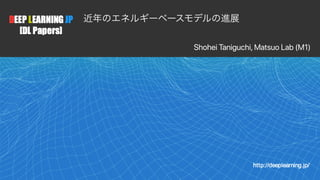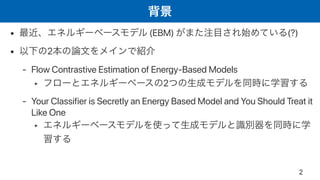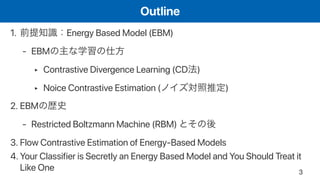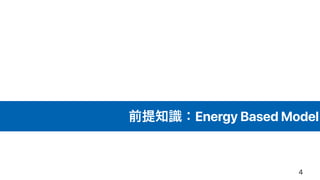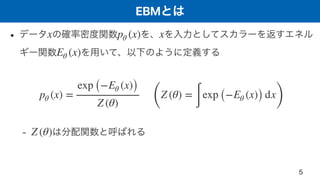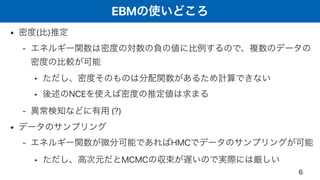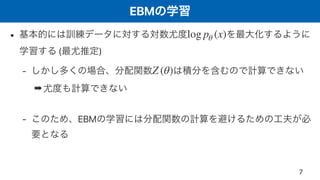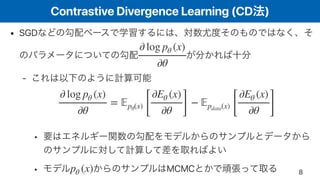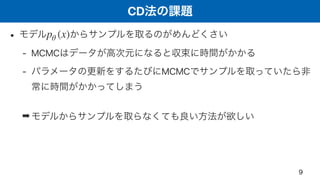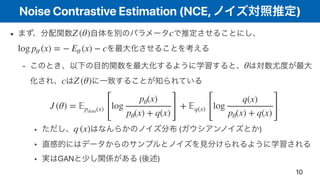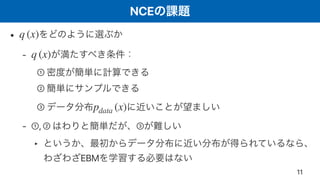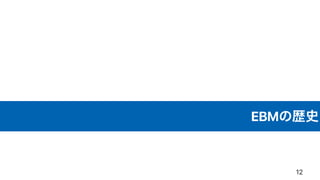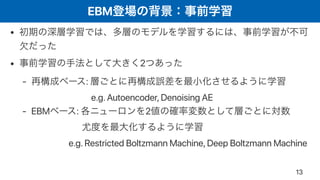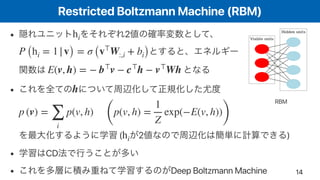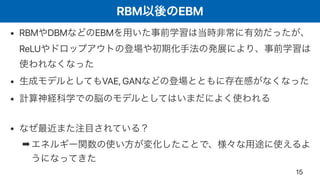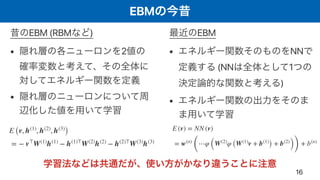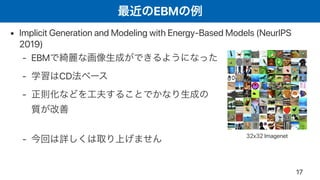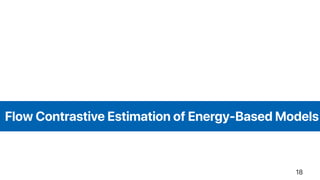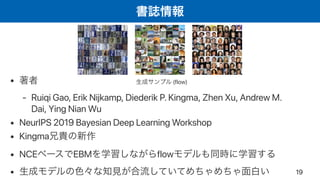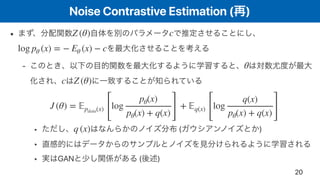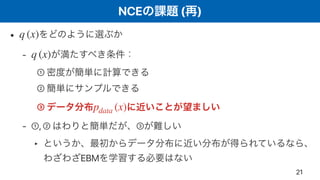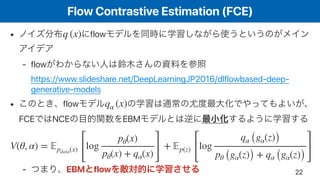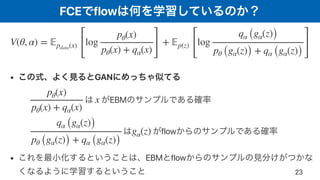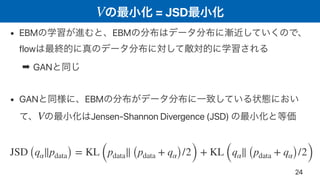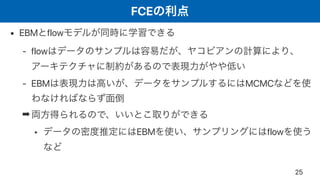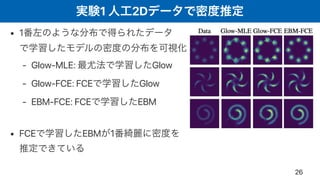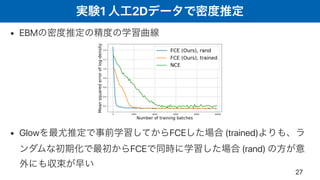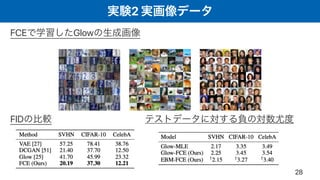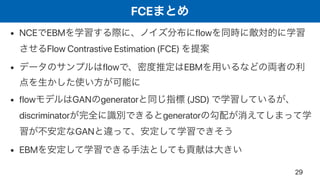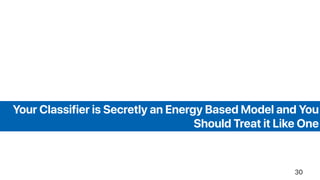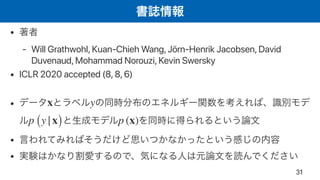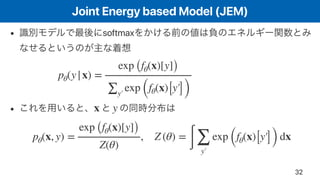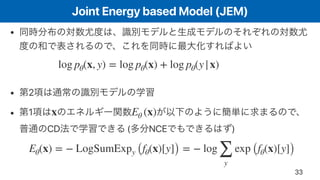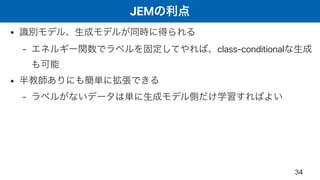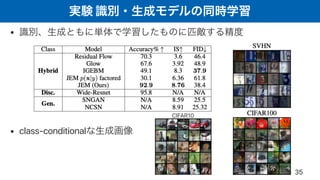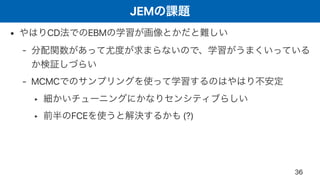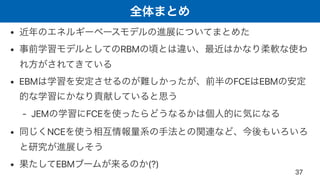1 de 37[DL輪読会]Flow-based Deep Generative ModelsDeep Learning JPPRML学習者から入る深層生成モデル入門tmtm otm【DL輪読会】ViT + Self Supervised LearningまとめDeep Learning JP【DL輪読会】Scaling Laws for Neural Language ModelsDeep Learning JP

### [DL輪読会]近年のエネルギーベースモデルの進展

1. 1 Shohei Taniguchi, Matsuo Lab (M1)
2. • (EBM) (?) • 2 - Flow Contrastive Estimation of Energy-Based Models ‣ 2 - Your Classifier is Secretly an Energy Based Model and You Should Treat it Like One ‣ 2
3. Outline 1. Energy Based Model (EBM) - EBM ‣ Contrastive Divergence Learning (CD ) ‣ Noice Contrastive Estimation ( ) 2. EBM - Restricted Boltzmann Machine (RBM) 3. Flow Contrastive Estimation of Energy-Based Models 4. Your Classifier is Secretly an Energy Based Model and You Should Treat it Like One 3
4. Energy Based Model 4
5. EBM • - x pθ (x) x Eθ (x) pθ (x) = exp (−Eθ (x)) Z (θ) ( Z (θ) = ∫ exp (−Eθ (x)) dx ) Z (θ) 5
6. EBM • ( ) - ‣ ‣ NCE - (?) • - HMC ‣ MCMC 6
7. EBM • ( ) - ➡ - EBM log pθ (x) Z (θ) 7
8. Contrastive Divergence Learning (CD ) • SGD - ‣ ‣ MCMC ∂ log pθ (x) ∂θ ∂ log pθ (x) ∂θ = 𝔼pθ(x) [ ∂Eθ (x) ∂θ ] − 𝔼pdata(x) [ ∂Eθ (x) ∂θ ] pθ (x) 8
9. CD • - MCMC - MCMC ➡ pθ (x) 9
10. Noise Contrastive Estimation (NCE, ) • - ‣ ( ) ‣ ‣ GAN ( ) Z (θ) c log pθ (x) = − Eθ (x) − c θ c Z (θ) J (θ) = 𝔼pdata(x) [ log pθ(x) pθ(x) + q(x)] + 𝔼q(x) [ log q(x) pθ(x) + q(x) ] q (x) 10
11. NCE • - ① ② ③ - ①, ② ③ ‣ EBM q (x) q (x) pdata (x) 11
12. EBM 12
13. EBM • • 2 - : e.g. Autoencoder, Denoising AE - EBM : 2 e.g. Restricted Boltzmann Machine, Deep Boltzmann Machine 13
14. Restricted Boltzmann Machine (RBM) • 2 • ( 2 ) • CD • Deep Boltzmann Machine hi P (hi = 1|v) = σ (v⊤ W:,i + bi) E(v, h) = − b⊤ v − c⊤ h − v⊤ Wh h p (v) = ∑ i p(v, h) ( p(v, h) = 1 Z exp(−E(v, h)) ) hi 14 RBM
15. RBM EBM • RBM DBM EBM ReLU • VAE, GAN • • ➡ 15
16. EBM EBM (RBM ) • 2 • EBM • NN (NN 1 ) • 16 E (v) = NN (v) = w(n) ( ⋯φ (W(2) φ (W(1) v + b(1) ) + b(2) )) + b(n) E (v, h(1) , h(2) , h(3) ) = − v⊤ W(1) h(1) − h(1)⊤ W(2) h(2) − h(2)⊤ W(3) h(3)
17. EBM • Implicit Generation and Modeling with Energy-Based Models (NeurIPS 2019) - EBM - CD - - 17 32x32 Imagenet
18. Flow Contrastive Estimation of Energy-Based Models 18
19. • - Ruiqi Gao, Erik Nijkamp, Diederik P. Kingma, Zhen Xu, Andrew M. Dai, Ying Nian Wu • NeurIPS 2019 Bayesian Deep Learning Workshop • Kingma • NCE EBM flow • 19 (flow)
20. Noise Contrastive Estimation ( ) • - ‣ ( ) ‣ ‣ GAN ( ) Z (θ) c log pθ (x) = − Eθ (x) − c θ c Z (θ) J (θ) = 𝔼pdata(x) [ log pθ(x) pθ(x) + q(x)] + 𝔼q(x) [ log q(x) pθ(x) + q(x) ] q (x) 20
21. NCE ( ) • - ① ② ③ - ①, ② ③ ‣ EBM q (x) q (x) pdata (x) 21
22. Flow Contrastive Estimation (FCE) • flow - flow https://www.slideshare.net/DeepLearningJP2016/dlflowbased-deep- generative-models • flow FCE NCE EBM - EBM flow q (x) qα (x) V(θ, α) = 𝔼pdata(x) [ log pθ(x) pθ(x) + qα(x)] + 𝔼p(z) [ log qα (gα(z)) pθ (gα(z)) + qα (gα(z)) ] 22
23. FCE flow • GAN EBM flow • EBM flow V(θ, α) = 𝔼pdata(x) [ log pθ(x) pθ(x) + qα(x) ] + 𝔼p(z) [ log qα (gα(z)) pθ (gα(z)) + qα (gα(z)) ] pθ(x) pθ(x) + qα(x) x qα (gα(z)) pθ (gα(z)) + qα (gα(z)) gα(z) 23
24. = JSDV • EBM EBM flow ➡ GAN • GAN EBM Jensen-Shannon Divergence (JSD)V JSD (qα∥pdata) = KL (pdata∥ (pdata + qα)/2) + KL (qα∥ (pdata + qα)/2) 24
25. FCE • EBM flow - flow - EBM MCMC ➡ ‣ EBM flow 25
26. 1 2D • 1 - Glow-MLE: Glow - Glow-FCE: FCE Glow - EBM-FCE: FCE EBM • FCE EBM 1 26
27. 1 2D • EBM • Glow FCE (trained) FCE (rand) 27
28. 2 FCE Glow FID 28
29. FCE • NCE EBM flow Flow Contrastive Estimation (FCE) • flow EBM • flow GAN generator (JSD) discriminator generator GAN • EBM 29
30. Your Classifier is Secretly an Energy Based Model and You Should Treat it Like One 30
31. • - Will Grathwohl, Kuan-Chieh Wang, Jörn-Henrik Jacobsen, David Duvenaud, Mohammad Norouzi, Kevin Swersky • ICLR 2020 accepted (8, 8, 6) • • • x y p (y|x) p (x) 31
32. Joint Energy based Model (JEM) • softmax • pθ(y|x) = exp (fθ(x)[y]) ∑y′ exp (fθ(x)[y′]) x y pθ(x, y) = exp (fθ(x)[y]) Z(θ) , Z (θ) = ∫ ∑ y′ exp (fθ(x)[y′]) dx 32
33. Joint Energy based Model (JEM) • • 2 • 1 CD ( NCE ) log pθ(x, y) = log pθ(x) + log pθ(y|x) x Eθ (x) Eθ(x) = − LogSumExpy (fθ(x)[y]) = − log ∑ y exp (fθ(x)[y]) 33
34. JEM • - class-conditional • - 34
35. • • class-conditional 35 CIFAR10
36. JEM • CD EBM - - MCMC ‣ ‣ FCE (?) 36
37. • • RBM • EBM FCE EBM - JEM FCE • NCE • EBM (?) 37